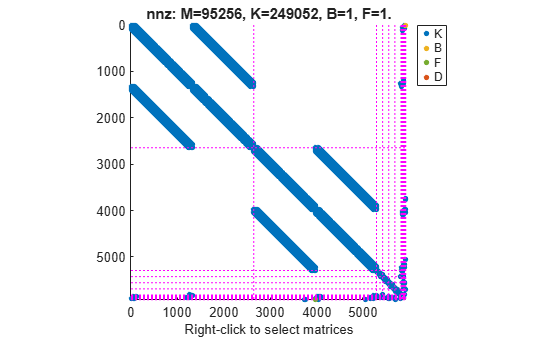# interface

Specify physical connections between components of `mechss` model

## Syntax

``sysCon = interface(sys,C1,IC1,C2,IC2)``
``sysCon = interface(sys,C,IC)``
``sysCon = interface(___,KI,CI)``

## Description

example

````sysCon = interface(sys,C1,IC1,C2,IC2)` specifies physical couplings between components `C1` and `C2` in the second-order sparse model `sys`. `IC1` and `IC2` contain the indices of the coupled degrees of freedom (DOF) relative to the DOFs of `C1` and `C2`. The physical interface is assumed rigid and satisfies the standard consistency and equilibrium conditions. `sysCon` is the resultant model with the specified physical connections. Use `showStateInfo` to get the list of all available components of `sys`.```

example

````sysCon = interface(sys,C,IC)` specifies that component `C` interfaces with the ground. Connecting the degrees of freedom q of `C` to the ground amounts to the zero displacement constraint `q(IC) = 0`.```
````sysCon = interface(___,KI,CI)` further specifies the stiffness `KI` and damping `CI` for nonrigid interfaces.```

## Examples

collapse all

For this example, consider a structural model that consists of two square plates connected with pillars at each vertex as depicted in the figure below. The lower plate is attached rigidly to the ground while the pillars are attached rigidly to each vertex of the square plate.Load the finite element model matrices contained in `platePillarModel.mat` and create the sparse second-order model representing the above system.

```load('platePillarModel.mat') sys = ... mechss(M1,[],K1,B1,F1,'Name','Plate1') + ... mechss(M2,[],K2,B2,F2,'Name','Plate2') + ... mechss(Mp,[],Kp,Bp,Fp,'Name','Pillar3') + ... mechss(Mp,[],Kp,Bp,Fp,'Name','Pillar4') + ... mechss(Mp,[],Kp,Bp,Fp,'Name','Pillar5') + ... mechss(Mp,[],Kp,Bp,Fp,'Name','Pillar6');```

Use `showStateInfo` to examine the components of the `mechss` model object.

`showStateInfo(sys)`
```The state groups are: Type Name Size ---------------------------- Component Plate1 2646 Component Plate2 2646 Component Pillar3 132 Component Pillar4 132 Component Pillar5 132 Component Pillar6 132 ```

Now, load the interfaced degrees of freedom (DOF) index data from `dofData.mat` and use `interface` to create the physical connections between the two plates and the four pillars. `dofs` is a `6x7` cell array where the first two rows contain DOF index data for the first and second plates while the remaining four rows contain index data for the four pillars.

```load('dofData.mat','dofs') for i=3:6 sys = interface(sys,"Plate1",dofs{1,i},"Pillar"+i,dofs{i,1}); sys = interface(sys,"Plate2",dofs{2,i},"Pillar"+i,dofs{i,2}); end```

Specify connection between the bottom plate and the ground.

`sysCon = interface(sys,"Plate2",dofs{2,7});`

Use `showStateInfo` to confirm the physical interfaces.

`showStateInfo(sysCon)`
```The state groups are: Type Name Size ----------------------------------- Component Plate1 2646 Component Plate2 2646 Component Pillar3 132 Component Pillar4 132 Component Pillar5 132 Component Pillar6 132 Interface Plate1-Pillar3 12 Interface Plate2-Pillar3 12 Interface Plate1-Pillar4 12 Interface Plate2-Pillar4 12 Interface Plate1-Pillar5 12 Interface Plate2-Pillar5 12 Interface Plate1-Pillar6 12 Interface Plate2-Pillar6 12 Interface Plate2-Ground 6 ```

You can use `spy` to visualize the sparse matrices in the final model.

`spy(sysCon)`The data set for this example was provided by Victor Dolk from ASML.

## Input Arguments

collapse all

Sparse second-order model, specified as a `mechss` model object. For more information, see `mechss`.

Components of `sys` to connect, specified as a string or an array of character vectors. Use `showStateInfo` to get the list of all available components of `sys`.

Index information of components to connect, specified as an `Nc`-by-`Ni` cell array, where `Nc` is the number of components and `Ni` is the number of physical interfaces.

Stiffness matrix, specified as an `Nq`-by-`Nq` sparse matrix, where `Nq` is the number of DOFs in `sys`.

Damping matrix, specified as an `Nq`-by-`Nq` sparse matrix, where `Nq` is the number of DOFs in `sys`.

## Output Arguments

collapse all

Output system with physical interfaces, returned as a `mechss` model object. Use `showStateInfo` to examine the list of physical interfaces in the system.

## Algorithms

Dual Assembly

`interface` uses the concept of dual assembly to physically connect the degrees of freedom (DOF) of the model components. For `n` substructures in the physical domain, the sparse matrices in block diagonal form are:

where, f is the force vector dependent on time and g is the vector of internal forces at the interface.

Two interfaced components share a set of DOFs in the global finite element mesh q: the subset N1 of DOFs from the first component coincides with the subset N2 of DOFs from the second component. The coupling between the two components is rigid only if:

• The displacements q at the shared DOFs are the same for both components.

`$q\left({N}_{1}\right)=q\left({N}_{2}\right)$`

• The forces g one component exerts on the other are opposite (by the action/reaction principle).

`$g\left({N}_{1}\right)+g\left({N}_{2}\right)=0$`

This relation can be summarized as:

where, H is a localisation matrix with entries 0, 1, or -1. The equation Hq = 0 is equivalent to q(N1) = q(N2), and the equation g = −HT λ is equivalent to g(N1) = −λ and g(N2) = λ. These equations can be combined in the differential-algebraic equation (DAE) form:

`$\begin{array}{l}\left[\begin{array}{cc}M& 0\\ 0& 0\end{array}\right]\left[\begin{array}{c}\stackrel{¨}{q}\\ \stackrel{¨}{\lambda }\end{array}\right]+\left[\begin{array}{cc}C& 0\\ 0& 0\end{array}\right]\left[\begin{array}{c}\stackrel{˙}{q}\\ \stackrel{˙}{\lambda }\end{array}\right]+\left[\begin{array}{cc}K& {H}^{T}\\ H& 0\end{array}\right]\left[\begin{array}{c}q\\ \lambda \end{array}\right]=\left[\begin{array}{c}B\\ 0\end{array}\right]u\\ y=\left[\begin{array}{cc}F& 0\end{array}\right]\left[\begin{array}{c}q\\ \lambda \end{array}\right]+\left[\begin{array}{cc}G& 0\end{array}\right]\left[\begin{array}{c}\stackrel{˙}{q}\\ \stackrel{˙}{\lambda }\end{array}\right]+Du\end{array}$`

This DAE model is called the dual assembly model of the overall structure. While the principle was explained for two components, this model can accommodate multiple interfaces, including interfaces involving more than two components.

Nonrigid interface

In non-rigid interfaces, the displacements q1(N1) and q2(N2) are allowed to differ and the internal force is given by:

This models spring-damper-like connections between DOFs N1 in the first component and DOFs N2 in the second component. Going from rigid to non-rigid connection eliminates the algebraic constraints Hq = 0 and explicitates the internal forces. Then, eliminate λ to obtain:

This is the set of the primal-assembly equations for non-rigid coupling form that remains symmetric when the uncoupled model is symmetric. A drawback of this form is that the coupling terms ${H}^{T}{C}_{i}H$ and ${H}^{T}{K}_{i}H$ may cause fill-in. To avoid this, `interface` instead constructs the dual-assembly form:

`$\left[\begin{array}{ccc}M& 0& 0\\ 0& 0& 0\\ 0& 0& 0\end{array}\right]\left[\begin{array}{c}\stackrel{¨}{q}\\ \stackrel{¨}{\delta }\\ \stackrel{¨}{\lambda }\end{array}\right]+\left[\begin{array}{ccc}C& 0& 0\\ 0& {C}_{i}& 0\\ 0& 0& 0\end{array}\right]\left[\begin{array}{c}\stackrel{˙}{q}\\ \stackrel{˙}{\delta }\\ \stackrel{˙}{\lambda }\end{array}\right]+\left[\begin{array}{ccc}K& 0& {H}^{T}\\ 0& {K}_{i}& -I\\ H& -I& 0\end{array}\right]\left[\begin{array}{c}q\\ \delta \\ \lambda \end{array}\right]=\left[\begin{array}{c}B\\ 0\\ 0\end{array}\right]u$`